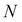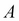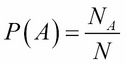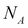•#### Learning Bayesian Models with R#### Overview of this book

Learning Bayesian Models with RCreditswww.PacktPub.comPrefaceFree Chapter
Introducing the Probability TheoryThe R EnvironmentIntroducing Bayesian InferenceMachine Learning Using Bayesian InferenceBayesian Regression ModelsBayesian Classification ModelsBayesian Models for Unsupervised LearningBayesian Neural NetworksBayesian Modeling at Big Data ScaleIndex## Chapter 1. Introducing the Probability Theory

Bayesian inference is a method of learning about the relationship between variables from data, in the presence of uncertainty, in real-world problems. It is one of the frameworks of probability theory. Any reader interested in Bayesian inference should have a good knowledge of probability theory to understand and use Bayesian inference. This chapter covers an overview of probability theory, which will be sufficient to understand the rest of the chapters in this book.

It was Pierre-Simon Laplace who first proposed a formal definition of probability with mathematical rigor. This definition is called the Classical Definition and it states the following:

 The theory of chance consists in reducing all the events of the same kind to a certain number of cases equally possible, that is to say, to such as we may be equally undecided about in regard to their existence, and in determining the number of cases favorable to the event whose probability is sought. The ratio of this number to that of all the cases possible is the measure of this probability, which is thus simply a fraction whose numerator is the number of favorable cases and whose denominator is the number of all the cases possible. --Pierre-Simon Laplace, A Philosophical Essay on Probabilities

What this definition means is that, if a random experiment can result inmutually exclusive and equally likely outcomes, the probability of the eventis given by:Here,is the number of occurrences of the event.

To illustrate this concept, let us take a simple example of a rolling dice. If the dice is a fair dice, then all the faces will have an equal chance of showing up when the dice is rolled. Then, the probability of each face showing up is 1/6. However, when one rolls the dice 100 times, all the faces will not come in equal proportions of 1/6 due to random fluctuations. The estimate of probability of each face is the number of times the face shows up divided by the number of rolls. As the denominator is very large, this ratio will be close to 1/6.

In the long run, this classical definition treats the probability of an uncertain event as the relative frequency of its occurrence. This is also called a frequentist approach to probability. Although this approach is suitable for a large class of problems, there are cases where this type of approach cannot be used. As an example, consider the following question: Is Pataliputra the name of an ancient city or a king? In such cases, we have a degree of belief in various plausible answers, but it is not based on counts in the outcome of an experiment (in the Sanskrit language Putra means son, therefore some people may believe that Pataliputra is the name of an ancient king in India, but it is a city).

Another example is, What is the chance of the Democratic Party winning the election in 2016 in America? Some people may believe it is 1/2 and some people may believe it is 2/3. In this case, probability is defined as the degree of belief of a person in the outcome of an uncertain event. This is called the subjective definition of probability.

One of the limitations of the classical or frequentist definition of probability is that it cannot address subjective probabilities. As we will see later in this book, Bayesian inference is a natural framework for treating both frequentist and subjective interpretations of probability.